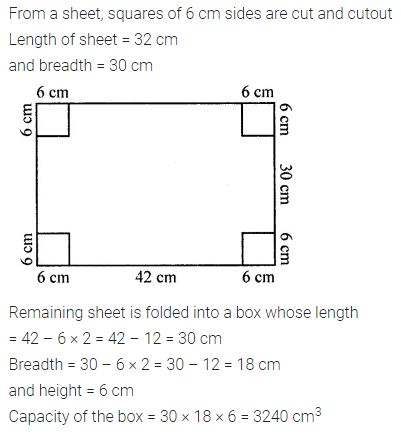## ML Aggarwal Class 8 Solutions for ICSE Maths Chapter 18 Mensuration Objective Type Questions

Mental Maths
Question 1.
Fill in the blanks:
(i) Area of a parallelogram = base × …….
(ii) Area of a trapezium = $$\frac{1}{2}$$ × ……….. × distance between parallel sides.
(iii) Area of a rhombus = $$\frac{1}{2}$$ × product of ……..
(iv) Area is measured in ……….. units.
(v) Volume of a solid is the measurement of ………… occupied by it.
(vi) Volume is measured in ………… units.
(vii) The volume of a unit cube is ……….
(viii) 1 litre = …………… cm3
(ix) 1 m3 = ………… litres
(x) Volume of a cuboid = ……….. × height.
(xi) Cylinders in which line segment joining the centres of the circular faces is perpendicular to the base are called ……….
(xii) Volume of a cylinder = area of base × ………..
(xiii) Area of four walls = perimeter of floor × …….
(xiv) Lateral surface area of a cube = 4 × (…………)2
(xv) Total surface area of a cylinder of radius r and height h is ………..
Solution: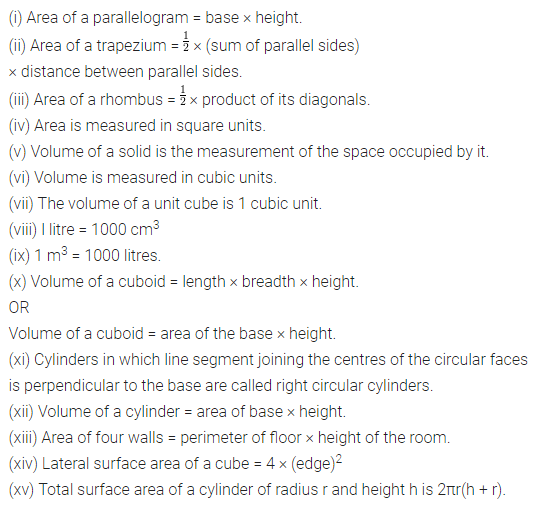Question 2.
State which of the following statements are true (T) or false (F):
(i) Perimeter of a rectangle is the sum of lengths of its four sides.
(ii) Area of a quadrilateral can be found by splitting it into two triangles.
(iiii) Perimeter of a circle of radius r = πr2.
(iv) Volume of a cube = 6 × (side)2
(v) 1 m3 = 100000 cm3
(vi) Total surface area of a cuboid
= 2 (lb + bh + hl)
(vii) There is no difference between volume and capacity.
(viii)Total surface area of a cylinder = lateral surface area + area of two circular ends.
(ix) Surface area of a cube = 4 × (side)2
(x) Lateral surface area of a cuboid = perimeter of base × height.
Solution: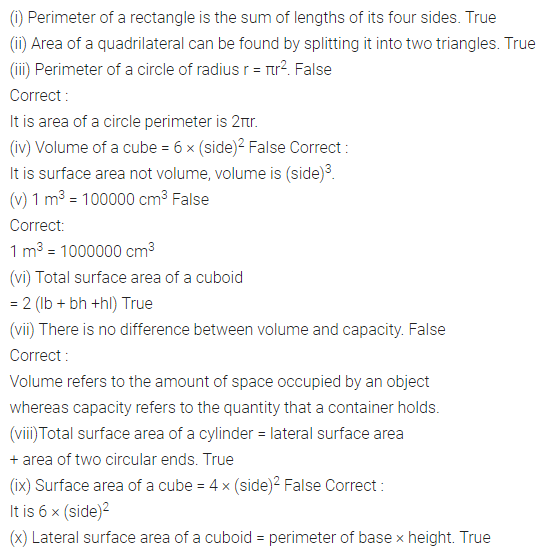Multiple Choice Questions
Choose the correct answer from the given four options (3 to 17):
Question 3.
Area of a triangle is 30 cm2. If its base is 10 cm, then its height is
(a) 5 cm
(b) 6 cm
(c) 7 cm
(d) 8 cm
Solution:Question 4.
If the perimeter of a square is 80 cm, then its area is
(a) 800 cm2
(b) 600 cm2
(c) 400 cm2
(d) 200 cm2
Solution: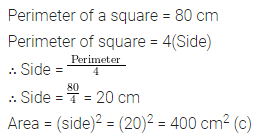Question 5.
Area of a parallelogram is 48 cm2. If its height is 6 cm then its base is
(a) 8 cm
(b) 4 cm
(c) 16 cm
(d) none of these
Solution: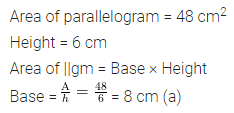Question 6.
If d is the diameter of a circle, then its area is
(a) πd2
(b) $$\frac{\pi d^{2}}{2}$$
(c) $$\frac{\pi d^{2}}{4}$$
(d) 2πd2
Solution:Question 7.
If the area of a trapezium is 64 cm2 and the distance between parallel sides is 8 cm, then sum of its parallel sides is
(a) 8 cm
(b) 4 cm
(c) 32 cm
(d) 16 cm
Solution: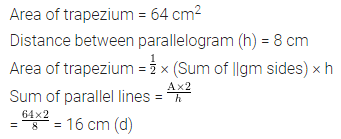Question 8.
Area of a rhombus whose diagonals are 8 cm and 6 cm is
(a) 48 cm2
(b) 24 cm2
(c) 12 cm2
(d) 96 cm2
Solution: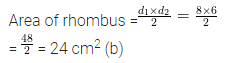Question 9.
If the lengths of diagonals of a rhombus is doubled, then area of rhombus will be
(a) doubled
(b) tripled
(c) four times
(d) remains same
Solution:Question 10.
If the length of a diagonal of a quadrilateral is 10 cm and lengths of the perpendiculars on it from opposite vertices are 4 cm and 6 cm, then area of quadrilateral is
(a) 100 cm2
(b) 200 cm2
(c) 50 cm2
(d) none of these
Solution: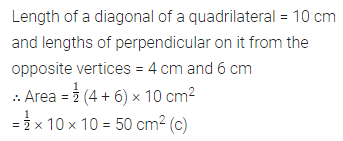Question 11.
Area of a rhombus is 90 cm2. If the length of one diagonal is 10 cm then the length of other diagonal is
(a) 18 cm
(b) 9 cm
(c) 36 cm
(d) 4.5 cm
Solution: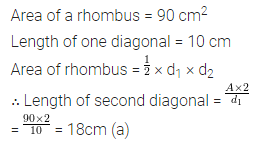Question 12.
If the volume of a cube is 729 cm3, then its surface area is
(a) 486 cm2
(b) 324 cm2
(c) 162 cm2
(d) none of these
Solution: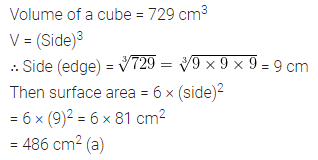Question 13.
If the lateral surface area of a cube is 100 cm2, then its volume is
(a) 25 cm3
(b) 125 cm3
(c) 625 cm3
(d) none of these
Solution: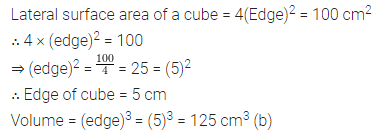Question 14.
If the length of side of a cube is doubled, then the ratio of volumes of new cube and original cube is
(a) 1 : 2
(b) 2 : 1
(c) 4 : 1
(d) 8 : 1
Solution: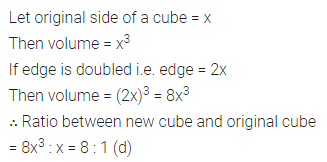Question 15.
If the dimensions of a rectangular room are 10m × 12m × 9m, then the cost of painting its four walls at the rate of ₹8 per m2 is
(a) ₹3186
(b) ₹3618
(c) ₹3168
(d) none of these
Solution:Question 16.
Volume of a cylinder is 1848 cm2. If the diameter of its base is 14 cm, then the height of the cylinder is
(a) 12 cm
(b) 6 cm
(c) 3 cm
(d) none of these
Solution:Question 17.
If the radius of a cylinder is doubled and height is halved, then new volume is
(a) same
(b) 2 times
(c) 4 times
(d) 8 times
Solution: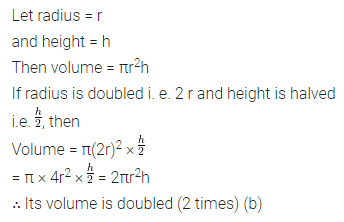Value Based Questions
Question 1.
Pulkit painted four walls and roof of a rectangular room of size 10m × 12m × 12m. He got ₹10 per m2 for his work. How much money did he earn? He always gives one-fourth of his income to an orphanage. Find how much money he gave to the orphanage? What values are being promoted?
Solution: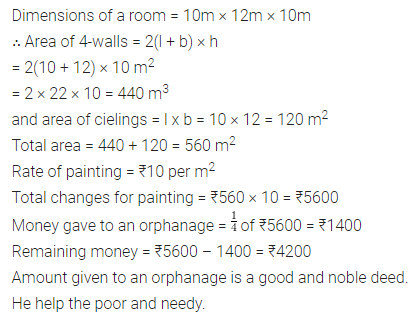Question 2.
In a slogan writing competition in a school, Rama wrote the slogan ‘Truth pays, never betrays’ on a trapezium shaped cardboard. If the lengths of parallel sides of trapezium are 60 cm and 80 cm and the distance between them is 50 cm, find the area of trapezium. What are the advantages of speaking truth?
Solution: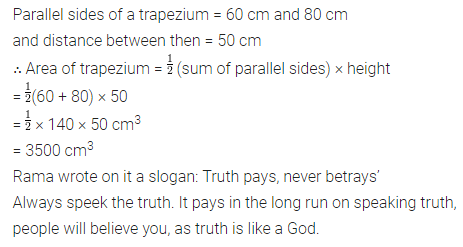Higher Order Thinking Skills (Hots)
Question 1.
The length of a room is 50% more than its breadth. The cost of carpeting the room at the rate of ₹38.50 m2 is ₹924 and the cost of papering the walls at ₹3.30 m2 is ₹214.50. If the room has one door of dimensions 1 m × 2 m and two windows each of dimensions 1 m × 1.5 m, find the dimensions of the room.
Solution: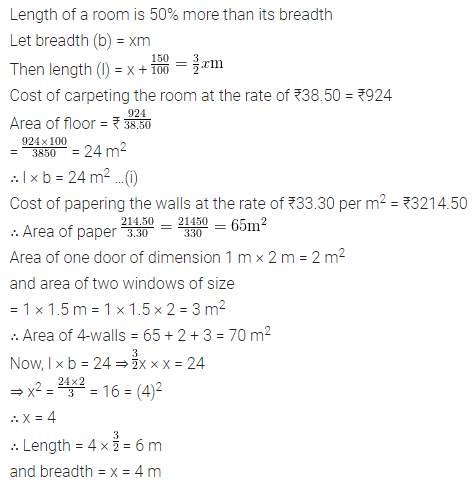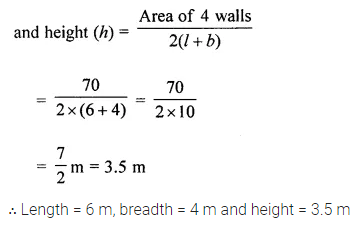Question 2.
The barrel of a fountain pen, cylindrical in shape, is 7 cm long and 5 mm in diameter. A full barrel of ink in the pen will be used up when writing 310 words on an average. How many words would use up a bottle of ink containing one-fifth of a litre? Answer correct to the nearest 100 words.
Solution: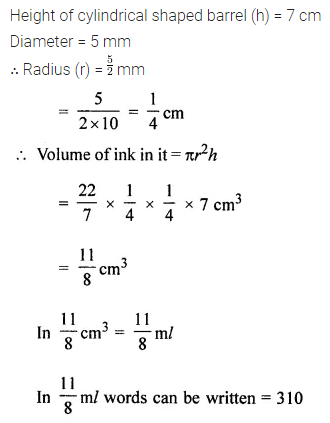Question 3.
A cylindrical jar is 20 cm high with an internal diameter 7 cm. An iron cube of edge 5 cm is immersed in the jar completely in the water which was originally 12 cm high. Find the rise in the level of water.
Solution:Question 4.
Squares each of side 6 cm are cut off from the four comers of a sheet of tin measuring 42 cm by 30 cm. The remaining portion of the tin sheet is made into an open box by folding up the flaps. Find the capacity of the box.
Solution: